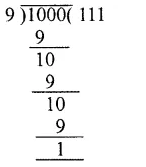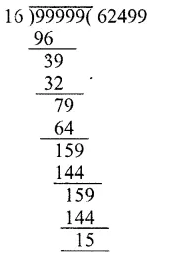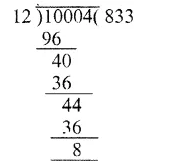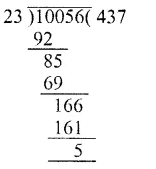## RS Aggarwal Class 6 Solutions Chapter 3 Whole Numbers Ex 3F

These Solutions are part of RS Aggarwal Solutions Class 6. Here we have given RS Aggarwal Solutions Class 6 Chapter 3 Whole Numbers Ex 3F.

Other Exercises

Objective questions
Mark against the correct answer in each of the following :

Question 1.
Solution:
The smallest whole number is 0 (b)

Question 2.
Solution:
The least 4-digit number = 1000
On dividing 1000 by 9, we get
Remainder = 1Least 4-digit number which is
Divisible by 9 = 1000 – 1 + 9
= 1000 + 8
= 1008 (d)

Question 3.
Solution:
The largest 6-digit number = 999999
On dividing by 16, we get
Remainder =15The greatest 6-digit number divisible by 16
= 999999 – 15
= 999984 (c)

Question 4.
Solution:
On dividing 10004 by 12, we get remainder = 88 is to be subtracted from 10004 (c)

Question 5.
Solution:
On dividing 10056 by 23 We get remainder =12The least number to be added = 23 – 5
= 18 (b)

Question 6.
Solution:
On dividing 457 by 11
We get remainder = 6Which is greater than half of 11
The number nearest to 457 which is divisible 11 will be = 457 – 6 + 11
= 457 + 5
= 462 (d)

Question 7.
Solution:
Whole number between 1018 and 1203 are 1019 to 1202 are 1202 – 1018
= 184 (c)

Question 8.
Solution:
Divisor = 46
Quotient =11
Remainder =15
Number = Divisor x Quotient + Remainder
= 46 x 11 + 15
= 506 + 15
= 521 (b)

Question 9.
Solution:
Dividend = 199
Quotient =16
Remainder = 7
Divisor = $$\\ \frac { 199-7 }{ 16 }$$ = $$\\ \frac { 192 }{ 16 }$$
= 12 (c)

Question 10.
Solution:
7589 – ? = 3434
Required number = 7589 – 3434
= 4155 (c)

Question 11.
Solution:
587 x 99 = 587 x (100 – 1)
= 587 x 100 – 587 x 1
= 58700 – 587
= 58113 (c)

Question 12.
Solution:
4 x 538 x 25 = 538 x 4 x 25
= 538 x 100
= 53800 (c)

Question 13.
Solution:
24679 x 92 + 24679 x 8
= 24679 x (92 + 8)
= 24679 x 100
= 2467900 (c)

Question 14.
Solution:
1625 x 1625 – 1625 x 625
= 1625 (1625 – 625)
= 1625 x 1000
= 1625000 (a)

Question 15.
Solution:
1568 x 185 – 1568 x 85
= 1568 (185 – 85)
= 1568 x 100
= 156800 (c)

Question 16.
Solution:
888 + 111 + 555 = 111 x ?
= 11 (8 + 7 + 5)
= 111 x 20 (c)

Question 17.
Solution:
The sum of two odd number is an even number (b)

Question 18.
Solution:
The product of two odd numbers is an odd number (a)

Question 19.
Solution:
If a is a whole number such that
a + a = a, then a = 0
as 0 + 0 = 0
None of these (d)

Question 20.
Solution:
The predecessor of 10000 is 10000 – 1
= 9999 (b)

Question 21.
Solution:
The successor of 1001 is 1001 + 1
= 1002 (b)

Question 22.
Solution:
The smallest even whole number is 2 (b)

Hope given RS Aggarwal Solutions Class 6 Chapter 3 Whole Numbers Ex 3F are helpful to complete your math homework.

If you have any doubts, please comment below. Learn Insta try to provide online math tutoring for you.